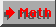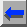# Heegner Points Package

Developed by Peter Green.

To install the Heegner Tools it is best if you have a Linux machine with PARI/GP (v.2.1.1 or higher) already installed. To download and install the Heegner point package, follow these steps.

Reconfiguring and recompiling.

Steps 1.1 - 1.4 are only needed if you do not run Linux/x86 and PARI 2.1. In that case these steps will reconfigure and recompile the cmtools.so plugin to fit your system. This supposes that your system support dlopen and shared libraries.

You need to have kept the directory when you have compiled PARI/GP. It is usually named pari or pari-2.1.x. We will suppose it is /usr/src/pari-2.1.3.

1.2. Save this file in the same directory as heegnertools.tgz and, from within this directory type

tar xzvf heegnertoolsmake.tgz
cd heegnertools/src

1.3. Link the directory when you have compiled PARI/GP to ./pari with ln -s /usr/src/pari-2.1.3 pari

1.4. Type

./configure
make clean
make all
cd ..

This step is finished. Do not be worried by all the warnings. The last line should look like (but not be exactly as)

/usr/bin/ld -shared -lc -o cmtools.so cremona.o heegner.o hpxh.o modsymb.o tate.o

2. From within the directory where you have stored heegnertools.tgz type

tar xzvf heegnertools.tgz

at the Unix prompt. This creates a new directory called heegnertools and completes the installation.

3. I have been told that the procedure above may be obsolete and does not always work. For those who encounter difficulties, the following letter by Utpal Sarkar might contain useful tips.

# 2. Using the Heegner Points package

1. Go into the directory heegnertools created in the installation process, and type

gp

at the Unix prompt. GP/PARI should start as usual.

2. The Heegner Tools are organized into components. If you type

?components

from the GP prompt, you will receive the message:

The following components have been loaded: cremona, heegner, hpxh, modsymb, tate. For more information about the component *, type ?*. For a list of loadable components type clist().

# 3. Summary of functions in the Heegner package

The different components of the Heegner package are comprised of the following functions.

### 1. The cremona component

ellcremona("NIk") returns the coefficient vector for the elliptic curve of conductor N, isogeny class I and isomorphism class k as listed in Cremona's tables; for instance ellcremona("37B2") returns [0,1,1,-1873,-31833]; the defaults are I = A and k = 1, and so to get the curve 37A1, it suffices to type ellcremona("37").

ellini(E,{p=0},{prec=0}) works like ellinit, but allows you to easily specify the prime at which to calculate the relevant data (default real), and the precision of that calculation (default is 0, in which case the current realprecision is used for real calculations); moreover, instead of a five-component vector for, the input E may be given in the form "NIk" as for ellcremona; for instance ellini("37") returns the data for the curve 37A1 over C to realprecision digits and ellini("11A2",11,25) returns the data for curve 11A2 over Q_11 to precision O(11^25).

### 2. The heegner component

elltautopoint(E,t) takes as input an elliptic curve E (the output of the function ellinit or ellini) and a point t in the complex upper half-plane H, and returns the corresponding point point P=[x,y] in E(C).

elltautoz(E,t) takes as input an elliptic curve E (the output of the function ellinit or ellini) and a point t in the upper half-plane H, and returns the corresponding point z in C/L, where L is the Neron lattice of E.

qfbclassgroup(delta) takes as input a discriminant delta (positive or negative) and returns a vector of length h (= class number) consisting of SL(2,Z)-representatives of the primitive binary quadratic forms of discriminant delta.

qfblift(F,N,s) takes as input a positive definite primitive integral binary quadratic form F, a positive integer N, and s a square root of discrim(F) mod N; returns an SL(2,Z)-equivalent quadratic form [A',B',C'] with A' = 0 (mod N) B' = s (mod N) and A' minimal.

ellHeegnerPoints(E,D,{s=0}) takes as input an elliptic curve E of conductor N, a discriminant D prime to N, and optionally an orientation s (i.e. a square root of D mod N); returns a vector consisting of h(D) Heegner points on the elliptic curve corresponding to the input data.

### 3. The hpxh component

hpxhint(tau1,tau2,z1,z2,table,prec) evaluates the double integral on Hp x H defined in
H. Darmon, Integration on Hp x H and arithmetic applications
on the region [tau1,tau2] x [z1,z2] to a precision of prec significant p-adic digits using the Manin symbols in table. At present, this function is only implemented for elliptic curves E of prime conductor p. Details of the implementation are given in
H. Darmon, P. Green, Elliptic curves and class fields of real quadratic fields: algorithms and evidence.

ellStarkHeegner(rawmininid,functional,F,Oprec) returns the Stark-Heegner period corresponding to the raw Manin table database entry rawminid (see the help for the modsymb package), the vector functional which is an element of the Z-dual of the complex period lattice written with respect to the real-imaginary period basis, the binary quadratic form F, and the precision Oprec written in the form O(p^k); for example, the call ellStarkHeegner("37A",[1,0],Qfb(1,6,-6),O(37^4)) yields the real period coefficient of the Stark-Heegner period for the isogeny class 37A (Cremona) and the point tau=-3+sqrt(15) generating the order of discriminat 60, calculated to a precision of 37^(-4).

### 4. The modsymb component

ellmaninraw(E) returns a table of raw Manin symbols corresponding to the elliptic curve E.

ellcuspint(table,a,b) computes the integral between cusps a and b of the modular function with Manin symbols table table (output of ellmaninraw).

ellmanindbadd(cremonaentry) stores in the Manin symbol database a table of raw Manin symbols corresponding to the isogeny class cremonaentry which should be expressed in the format explained in the help for the cremona component; for example ellmanindbadd("37A") stores in the database a table of raw Manin symbols for Cremona's isogeny class 37A.

ellmanindbget(cremonaentry) retrieves from the Manin symbol database a table of raw Manin symbols corresponding to the isogeny class cremonaentry which should be expressed in the format explained in the help for the cremona component; for example ellmanindbget("37A") retrieves from the database the table of raw Manin symbols for Cremona's isogeny class 37A.

### 5. The tate component

ellchangeofvars(E1,E2) finds the change of variables vector [u,r,s,t] between elliptic curves E1 and E2. When E1 and E2 are both p-adic, the vector entries may be in Cp.

elltate(E,c) computes the tate uniformization of the elliptic curve E at the the point c in Cp.

# 4. Sample Pari dialogues

### 1. Calculating classical Heegner points

/* let E be the curve y^2 + y = x^3 - x of conductor 37 */
> E = ellini("37A1")
%1 = [0, 0, 1, -1, 0, 0, -2, 1, -1, 48, -216, 37, 110592/37,
[0.8375654352833230354448108990, 0.2695944364054445582629379513,
-1.107159871688767593707748850]~, 2.993458646231959629832009979,
2.451389381986790060854224831*I, -0.4713192779568114758825938970,
-1.435456518668684318723208856*I, 7.338132740789576739070721003]

/* Compute the Heegner points of discriminant -28 of class number 1. */
> ellHeegnerPoints(E,-28)
%2 = [[1.999999999999999999999999999 - 5.04870979 E-29*I,
1.999999999999999999999999999 - 2.01948391 E-28*I]]

/* Compute Heegner points attached to the (non-fundamental discriminant
-28*9, of class number 4 */
> hp= ellHeegnerPoints(E,-28*9);
> length(hp)
%3 = 4

/* Compute characteristic polynomial of x-coordinates */
> prod(j=1,4, (t-hp[j]))
%4 = t^4 + (0.9999999999999999999999999984 + 1.62820890 E-27*I)*t^3
+ (102.0000000000000000000000000 + 1.17130067 E-26*I)*t^2
+ (-188.0000000000000000000000000 - 2.26182198 E-26*I)*t +
(84.99999999999999999999999997 + 9.69352279 E-27*I)

> h=round(%)
%7 = t^4 + t^3 + 102*t^2 - 188*t + 85

> polgalois(t^4 + t^3 + 102*t^2 - 188*t + 85)
%9 = [8, -1, 1]

### 2. Calculating Stark-Heegner points

/* real period coefficient of the Stark-Heegner period for the
isogeny class 37A and the point tau=-3+sqrt(15) generating the order
of discriminat 60, calculated to a precision of 37^(-4) */
> ellStarkHeegner("37A",[1,0],Qfb(1,6,-6),O(37^4))
[0, 1, 6/7]
Computing 2 integrals...
Computing integral 1...
musum : 0
Computing integral 2...
musum : 0
Modular symbol computation complete!
%1 = [24 + 13*37 + 4*37^3 + 19*37^4 + 2*37^5 + 36*37^6 + 31*37^7 + 8*37^8 +
O(37^9), 10 + 11*37 + 5*37^2 + 12*37^3 + 9*37^4 + 20*37^5 +
26*37^6 + 31*37^7 + 7*37^8 + O(37^9), 15]

/* compute the image under the Tate uniformisation of the
Stark-Heegner period to obtain a point on X0(37)+(C_37) */
> elltate(ellini("37A1",37,6),%)
%2 = [[24 + 14*37 + 33*37^2 + 36*37^3 + 13*37^4 + 5*37^5 + O(37^6), 17*37^4
+ 37^5 + O(37^6), 15], [26 + 7*37 + 7*37^2 + 9*37^4 + 26*37^5 +
O(37^6), 15*37^4 + 35*37^5 + O(37^6), 15]]

/* Observe this point agrees with the point on the curve
[2 + sqrt(3), -4 - sqrt(3)] to a precision of 37^-4 */